# Canburytech.net

## Drift alignment equations

### Apparent declination

We can use straightfoward vector analysis to derive an expression for the apparent declination of a star when viewed through a telescope with mis-aligned polar axis. Consider the following diagram, showing the position of a star, the direction of the true pole and the direction of the polar axis of a mis-aligned telescope mount. This diagram is drawn for the northern hemisphere.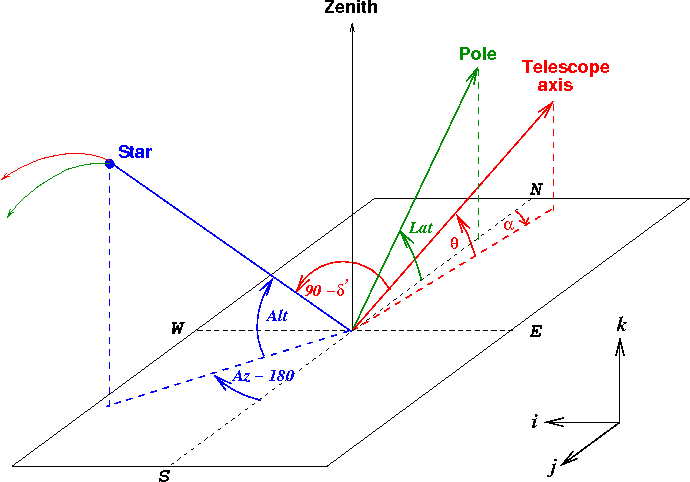The horizontal plane marked with the points of the compass represents the earth's surface at the location of the telescope. The true pole appears to be at an elevation of Lat, i.e. at an elevation equivalent to the observer's latitude. The telescope axis is at an azimuth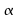and elevation. The star has an altitude Alt and an azimuth of Az (the actual azimuth is measured from the northerly direction, so the angle marked in the diagram is 180 degrees less than that). The angle between the telescope's polar axis and the star is 90 degrees minus the star's apparent declination, i.e. 90-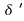, whereis the apparent declination. The star actually follows the path shown in green across the sky, but as observed by the mis-aligned telescope, the path appears as shown in red.

If we use the right-handed set of axes indicated in the diagram for the i,j and k coordinates and represent the directions of the star (s) and telescope axis (t) by vectors of unit length, then the direction of the star is given by:which reduces to: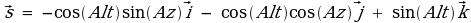and the direction of the telescope axis is given by: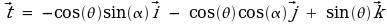Recalling that the dot product of two vectors is equal to the product of the magnitudes of the two vectors and the angle between them, we have: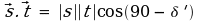Noting that: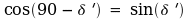and that the magnitudes of the s and t vectors are each equal to 1, the expression reduces to: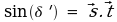Since: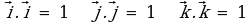and all other products involving i,j and k are zero, we obtain: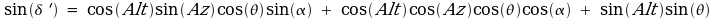or: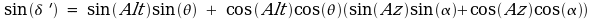This is our final equation for the apparent declination of the star. We need an expression involving the altitude and azimuth because we can adjust the altitude for the effects of refraction (this is discussed later). Note that if the telescope axis is perfectly aligned on the pole, thenis zero andequals Lat. In this case, the expression reduces to the standard equation for the declination of a star as a function of its altitude and azimuth, as obtained from spherical trigonometry.

### Apparent hour angle

The hour angle of a star is measured westwards (in the equatorial plane) from the point where the star crosses the local meridian. The apparent hour angle is defined here as being the angle measured westwards in the apparent equatorial plane from the apparent local meridian. The apparent equatorial plane is perpendicular to the telescope's mis-aligned polar axis, and the apparent meridian is located at an azimuth equal to the telescope axis azimuth, plus 180 degrees.

The appropriate angles are marked in the following diagram, using the same notation as before. Note that we are viewing this diagram from the north rather than the south - it's less confusing to illustrate the required angles that way.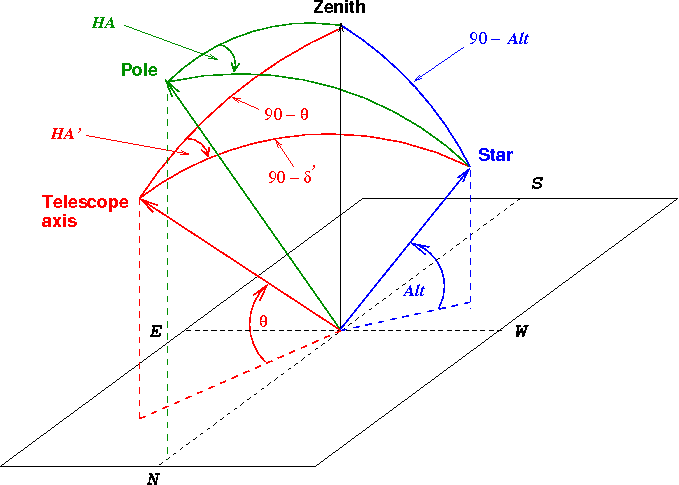The angle HA shown in green is the true hour angle of the star, and the angle HA' shown in red is the apparent hour angle. We can use the spherical cosine rule on the triangle formed by the telescope axis, the zenith and the star to obtain immediately: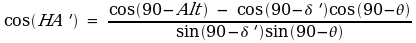which reduces to:### A simple approximation for declination drift rate

If the offset of the telescope axis from the pole is small, we can make the following simple approximation for the drift in declination: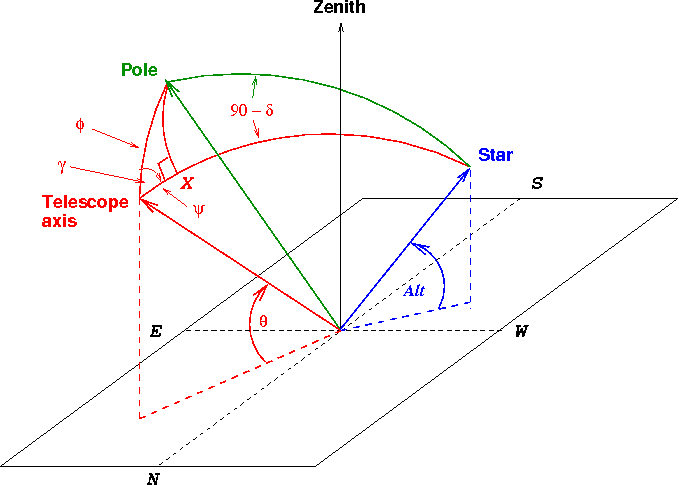The angular distance from the pole to the star is 90 degrees minus the true declination of the star. If we draw a great circle from the pole to the point X as shown, then the angular distance from X to the star is also 90 degrees minus the true declination of the star. But the total distance from the telescope axis to the star is 90 degrees minus the apparent declination of the star, so: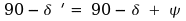The offset of the telescope axis from the pole is given by the angle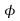; if this angle is small then the triangle formed by the pole, the telescope axis and X is approximately a planar right-angled triangle so we can say: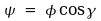For small values of, the rate of rotation of the star about the telescope axis will be nearly constant and equal to the sidereal rate, so after some re-arrangement: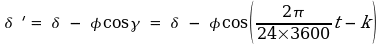where k is a constant and t is in seconds of time. The factors of 24 and 3600 allow for the fact that the angle varies throughradians in a day (a sidereal day is approximated as 24 hours).

Differentiating to obtain the declination drift rate, we have: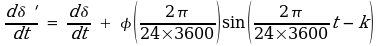The rate of variation of the true declination with time is zero, and the sin term has a maximum value of 1, so if we represent the maximum declination drift rate by MDR, then: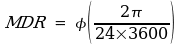or: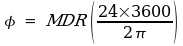So if we know the maximum rate of declination drift, we can estimate the maximum deviation of the telescope axis from the pole. (Typically we won't know for certain whether we have measured the maximum rate of drift because it varies round the sky; in that case all we can say is that the polar offset must be at least as big as the value that we deduce using this formula). In this expression, if the maximum drift rate is in arc seconds per second, then the angular offset from the pole will be in arc seconds, for example.

We can also use this formula the other way round to estimate the size of drift rate we have to measure to enable us to align the telescope axis within a desired distance from the pole.

### Size and direction of offset from pole

It is useful to have expressions for the size of the polar offset and its direction. Using the same symbols as in the above diagrams, we can apply the cosine rule to the triangle formed by the pole, the telescope axis and the zenith to obtain: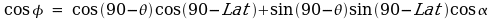which simplifies to: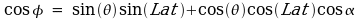whereis the elevation of the telescope axis, Lat is the observer's latitude andis the azimuth of the telescope axis.

In a similar way, using the same triangle, we obtain the following expression for the hour angle of the telescope axis:### Correction for atmospheric refraction

Atmospheric refraction has the effect of raising the apparent altitude of a star, with the size of the correction being greatest near the horizon. Since the stellar altitude appears in the equations for apparent declination and apparent hour angle, both of these quantities are affected by refraction in practice. Cassini's approximation can be used down to quite low altitudes and this formula is adopted here, where the apparent altitude Alt' is obtained by adding a correction to the true altitude Alt: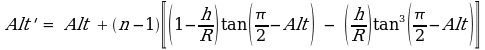In this expression, Alt' and Alt are both in radians. h is the height of the homogeneous atmosphere and R is the radius of the earth. The spreadsheet uses values of 7.99 km and 6370.9 km respectively. The refractive index of air is given by n; published expressions can be used for the dependence of refractive index on wavelength, temperature and pressure (and also humidity). A value of n = 1.0003 is typical.

The effects of refraction can become much more complicated close to the horizon, so the spreadsheet uses this formula from the zenith down to an altitude of 10 degrees, but then neglects the effects of refraction below that altitude.

### Order of calculation in the spreadsheet

The spreadsheet uses the exact equations to calculate the apparent drift in declination and hour angle over time, as follows:

1. Generate a series of Alt and Az values for a star as it moves across the sky.

2. If correcting for atmospheric refraction, calculate the new value of Alt' for each of the values of Alt above.

3. Use the appropriate value for the stellar altitude (Alt or Alt') in the expression for the apparent declination.

4. Calculate the declination drift as being the apparent declination minus the true declination (so a positive drift means a drift north and a negative drift means a drift south).

5. Use the calculated value of apparent declination and the appropriate value for the stellar altitude (Alt or Alt') in the expression for the apparent hour angle.

6. Calculate the hour angle drift by subtracting the constant change in hour angle due to the earth's rotation from the apparent hour angle, choosing the star's position on the true meridian as the arbitrary zero point. In this way, the hour angle drift is defined to be zero on the true meridian, whilst a positive drift means the star has drifted west and a negative drift means the star has drifted east relative to its expected location. If we centred a star in the eyepiece when on the true meridian and then followed it round the whole sky, we would expect to see an east/west drift, but the star would be centred again when on the true meridian.

In all these calculations (as always with trigonometry), we have to be careful that the angles that we calculate lie in the correct quadrant of the circle. We also need to take care that the appropriate values are used for the northern and southern hemispheres. The spreadsheet does this for you.